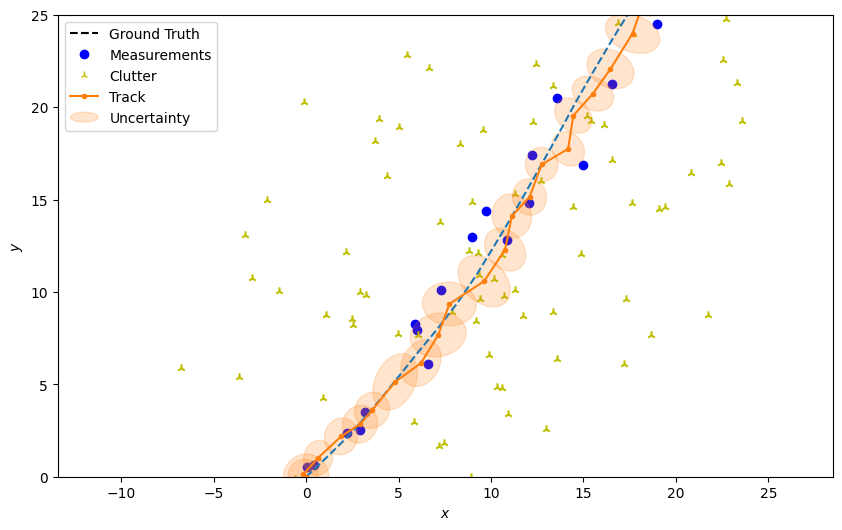# 7 - Probabilistic data association tutorial

Making an assignment between a single track and a single measurement can be problematic. In the previous tutorials you may have encountered the phenomenon of track seduction. This occurs when clutter, or other track, points are mis-associated with a prediction. If this happens repeatedly (as can be the case in high-clutter or low-$$p_d$$ situations) the track can deviate significantly from the truth.

Rather than make a firm assignment at each time-step, we could work out the probability that each measurement should be assigned to a particular target. We could then propagate a measure of these collective probabilities to mitigate the effect of track seduction.

Pictorially:

• Calculate a posterior for each hypothesis;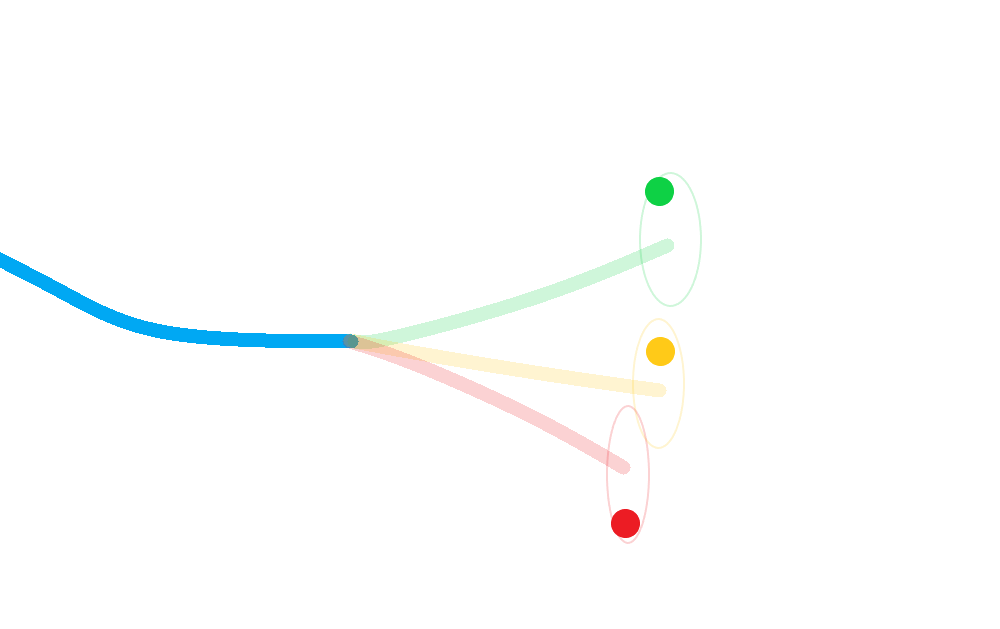• Weight each posterior state according to the probability that its corresponding hypothesis was true (including the probability of missed-detection);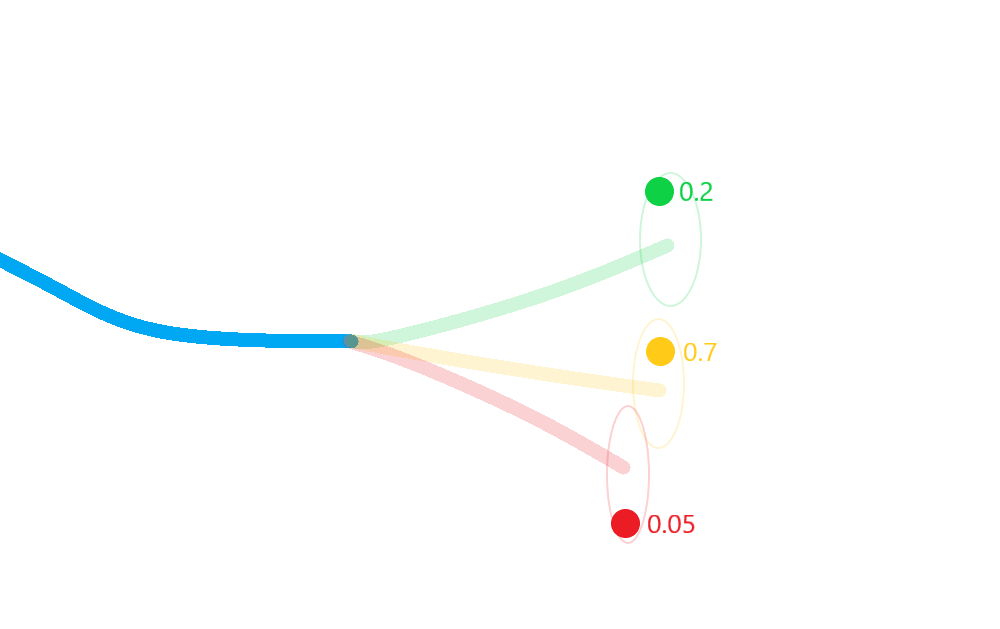• Merge the resulting estimate states in to a single posterior approximation.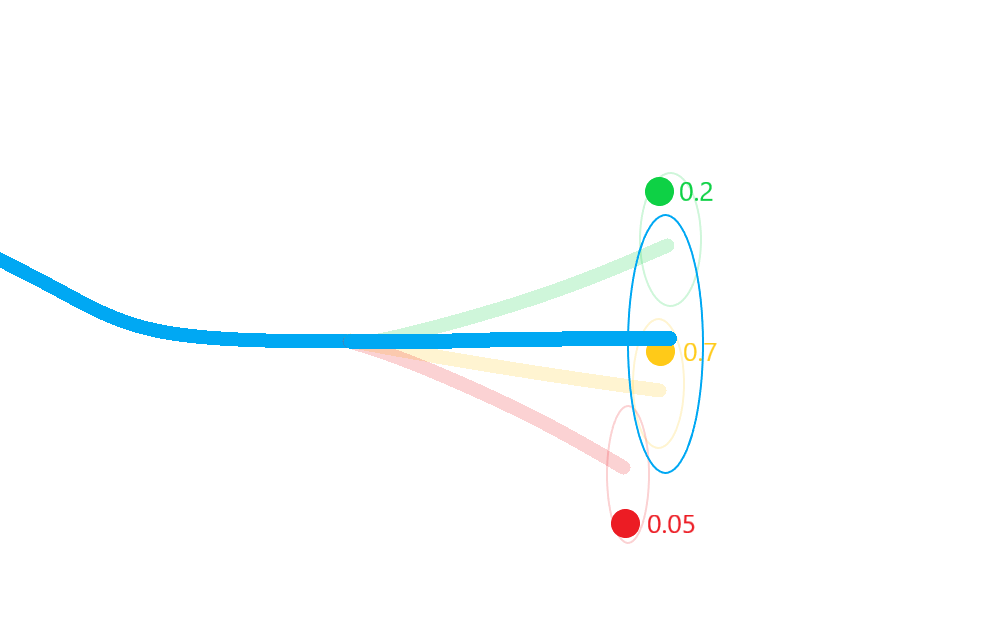This results in a more robust approximation to the posterior state covariances that incorporates not only the uncertainty in state, but also in the association.

## A PDA filter example

### Ground truth

So, as before, we’ll first begin by simulating some ground truth.

import numpy as np

from datetime import datetime
from datetime import timedelta

from stonesoup.models.transition.linear import CombinedLinearGaussianTransitionModel, \
ConstantVelocity
from stonesoup.types.groundtruth import GroundTruthPath, GroundTruthState

np.random.seed(1991)

start_time = datetime.now()
transition_model = CombinedLinearGaussianTransitionModel([ConstantVelocity(0.005),
ConstantVelocity(0.005)])
truth = GroundTruthPath([GroundTruthState([0, 1, 0, 1], timestamp=start_time)])
for k in range(1, 21):
truth.append(GroundTruthState(
transition_model.function(truth[k-1], noise=True, time_interval=timedelta(seconds=1)),
timestamp=start_time+timedelta(seconds=k)))


from scipy.stats import uniform

from stonesoup.types.detection import TrueDetection
from stonesoup.types.detection import Clutter
from stonesoup.models.measurement.linear import LinearGaussian
measurement_model = LinearGaussian(
ndim_state=4,
mapping=(0, 2),
noise_covar=np.array([[0.75, 0],
[0, 0.75]])
)

prob_detect = 0.9  # 90% chance of detection.

all_measurements = []
for state in truth:
measurement_set = set()

# Generate detection.
if np.random.rand() <= prob_detect:
measurement = measurement_model.function(state, noise=True)
groundtruth_path=truth,
timestamp=state.timestamp,
measurement_model=measurement_model))

# Generate clutter.
truth_x = state.state_vector
truth_y = state.state_vector
for _ in range(np.random.randint(10)):
x = uniform.rvs(truth_x - 10, 20)
y = uniform.rvs(truth_y - 10, 20)
measurement_model=measurement_model))

all_measurements.append(measurement_set)


Plot the ground truth and measurements with clutter.

from stonesoup.plotter import Plotter
plotter = Plotter()
plotter.ax.set_ylim(0, 25)
plotter.plot_ground_truths(truth, [0, 2])

# Plot true detections and clutter.
plotter.plot_measurements(all_measurements, [0, 2])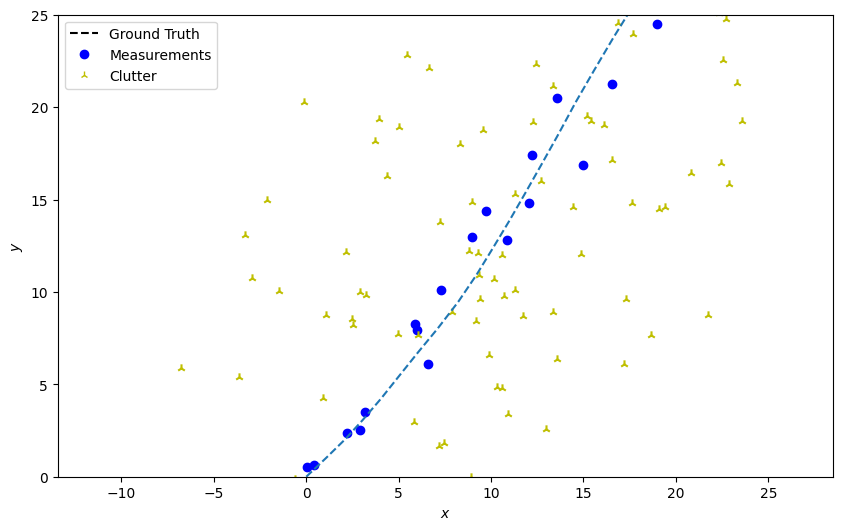Create the predictor and updater

from stonesoup.predictor.kalman import KalmanPredictor
predictor = KalmanPredictor(transition_model)

from stonesoup.updater.kalman import KalmanUpdater
updater = KalmanUpdater(measurement_model)


### Initialise Probabilistic Data Associator

The PDAHypothesiser and PDA associator generate track predictions and calculate probabilities for all prediction-detection pairs for a single prediction and multiple detections. The PDAHypothesiser returns a collection of SingleProbabilityHypothesis types. The PDA takes these hypotheses and returns a dictionary of key-value pairings of each track and detection which it is to be associated with.

from stonesoup.hypothesiser.probability import PDAHypothesiser
hypothesiser = PDAHypothesiser(predictor=predictor,
updater=updater,
clutter_spatial_density=0.125,
prob_detect=prob_detect)

from stonesoup.dataassociator.probability import PDA
data_associator = PDA(hypothesiser=hypothesiser)


### Run the PDA Filter

With these components, we can run the simulated data and clutter through the Kalman filter.

# Create prior
from stonesoup.types.state import GaussianState
prior = GaussianState([, , , ], np.diag([1.5, 0.5, 1.5, 0.5]), timestamp=start_time)

# Loop through the predict, hypothesise, associate and update steps.
from stonesoup.types.track import Track
from stonesoup.types.array import StateVectors  # For storing state vectors during association
from stonesoup.functions import gm_reduce_single  # For merging states to get posterior estimate
from stonesoup.types.update import GaussianStateUpdate  # To store posterior estimate

track = Track([prior])
for n, measurements in enumerate(all_measurements):
hypotheses = data_associator.associate({track},
measurements,
start_time + timedelta(seconds=n))

hypotheses = hypotheses[track]

# Loop through each hypothesis, creating posterior states for each, and merge to calculate
# approximation to actual posterior state mean and covariance.
posterior_states = []
posterior_state_weights = []
for hypothesis in hypotheses:
if not hypothesis:
posterior_states.append(hypothesis.prediction)
else:
posterior_state = updater.update(hypothesis)
posterior_states.append(posterior_state)
posterior_state_weights.append(
hypothesis.probability)

means = StateVectors([state.state_vector for state in posterior_states])
covars = np.stack([state.covar for state in posterior_states], axis=2)
weights = np.asarray(posterior_state_weights)

# Reduce mixture of states to one posterior estimate Gaussian.
post_mean, post_covar = gm_reduce_single(means, covars, weights)

# Add a Gaussian state approximation to the track.
track.append(GaussianStateUpdate(
post_mean, post_covar,
hypotheses,
hypotheses.measurement.timestamp))


Plot the resulting track

plotter.plot_tracks(track, [0, 2], uncertainty=True)
plotter.fig